# Examples of equations for 7th grade (12y)

#### Number of problems found: 529

• Large familyThe average age of all family members (children, mother, father, grandmother, grandfather) is 29 years. The average age of parents is 40 years, grandparents 66 years and all children are 5 years. How many children are there in this family?
• Boys and girlsThere are 28 girls in the hall. 5/7 of all children are boys. How many children and how many boys are there?
• FlourKim needs 3/4 cup of flour to make 12 cookies. How much flour would she need to make 60 cookies?
• RhombusIf we increase the rhombus side by 2.4 cm; its perimeter is 20 cm. How long was the side of the rhombus?
• Unknown number 5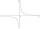Daniel think an integer. When he change this number at a ratio of 2:5 he got number 2.8. Determine what number think Daniel.
• Two ages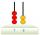Gabrielle is 9 years younger than Mikhail. The sum of their ages is 87. What is Mikhail's age?
• The quotientThe quotient of g and 55 is the same as 279. What is g?
• FriendsJames and Jaroslav are friends. Together they had 48 toy cars. But if John took 8 cars from Jaroslav, Jaroslav will be have 1.5 times more toy cars than James. How many toy cars had Jaroslav and James?
• Class 9.CProfessor collects money in the 9. C for a school trip. 2/3 of the collected amount was from girls and 1/4 from boys. The rest of 410 CZK went from the class fund. How much will the school trip cost in total?
• Fraction + eqSolve following simple equation with fractions: -5/6(8+5b) = 75 + 5/3b
• Four swords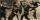Obelix has three helmets, four swords and five shields. How many swords must make at the blacksmith forge Metallurgix to be able to walk another 90 days in unique armor?
• Guess a fractionTom was asked to guess a fraction. The sum of 1/2 the numerator and 1/3 of its denominator is 30. If Tom subtracts 36 from its denominator, the fraction becomes 1/3. What is the fraction that Tom was asked to guess? (Leave your answer in simplest form)
• PlayingHow long have we trained on the pitch when we know that the warm-up took 10 minutes, we trained passes for one-third of the time and we played football half the time?
• Lumberjacks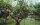Fifteen lumberjacks would take 12 days to destroy the broken trees. How many days would this work require if 5 workers added to it after 4 days?
• The tourist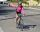The tourist came started from the hostel at an average speed of 5km/h. Half an hour later, the bicyclist started along the same route at a speed of 20km/h. How many minutes will a cyclist catch up and how many kilometers will he go?
• Tractors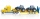5 tractors will plow the field for 8 hours. In 2 hours, 1 tractor broke down. How long do the remaining tractors complete the plow?
• In and out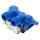The empty tank is filled in 12 minutes and empty in 16 minutes. How long does it take to fill if we forgot to close the drain? If the tank has 1000 l.
• Two trains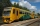From station A, the freight train traveled at a speed of 40 km/h in 9h. When he drove 15km, the fast train started from station A in the same direction at a speed of 70km/h. When will it the freight train catch up?
• SavingsEva borrowed 1/3 of her savings to her brother, 1/2 of savings spent in the store and 7 euros left. How much did she save?
• Negative in equation2x + 3 + 7x = – 24, what is the value of x?

Do you have an interesting mathematical word problem that you can't solve it? Submit a math problem, and we can try to solve it.

We will send a solution to your e-mail address. Solved examples are also published here. Please enter the e-mail correctly and check whether you don't have a full mailbox.

Please do not submit problems from current active competitions such as Mathematical Olympiad, correspondence seminars etc...

Do you have a linear equation or system of equations and looking for its solution? Or do you have quadratic equation?